Sorry, your browser does not support JavaScript!Home >

# Overview of Solar PV system monitoring

If you have a solar PV system, you can monitor it on Iammeter by installing our WiFi energy meters. IAMMETER displays different overview webpages for Solar PV System and Non-solar PV System.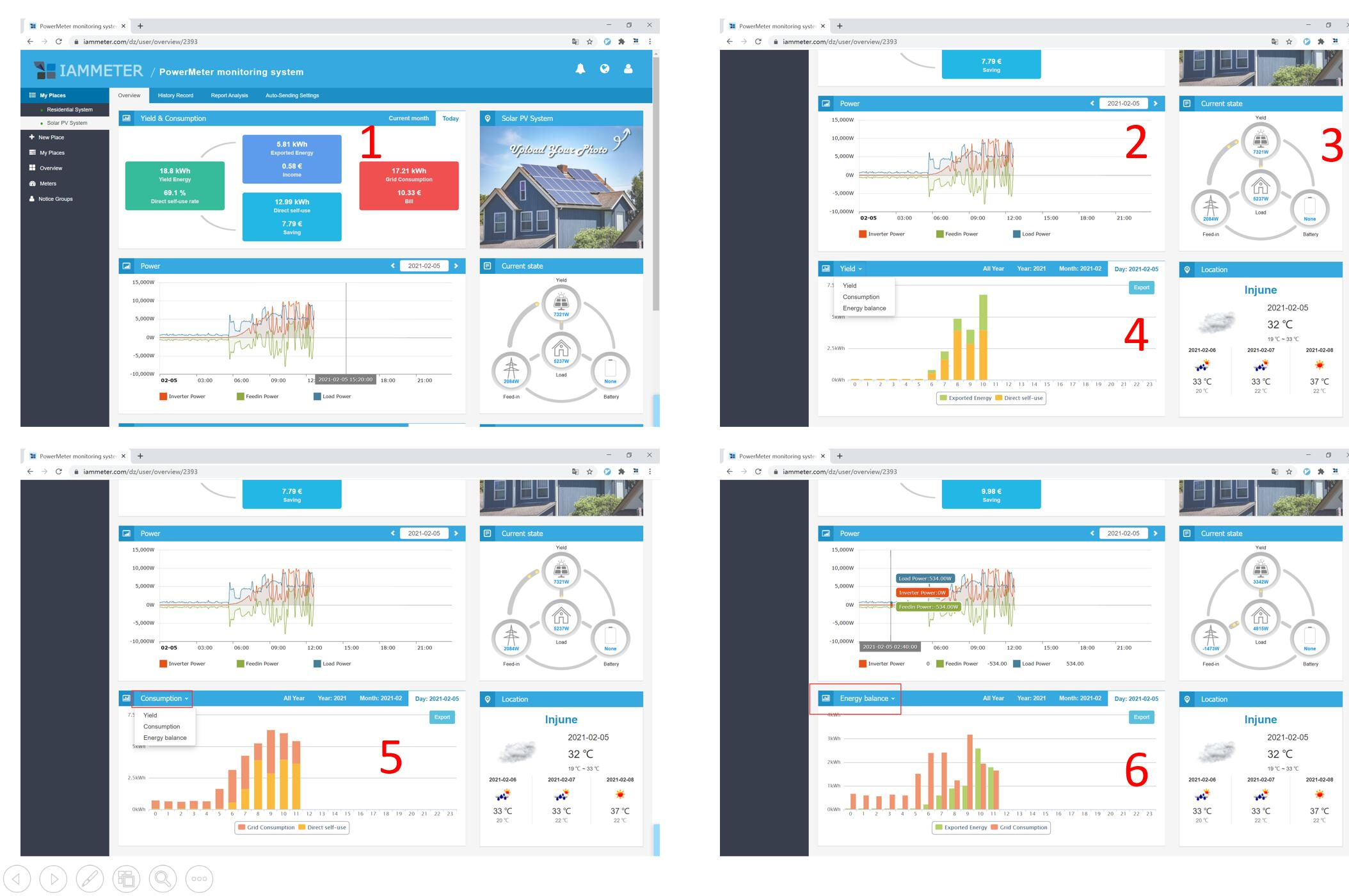# 1 Yield and consumption

This graph shows the KPIs of your solar PV system.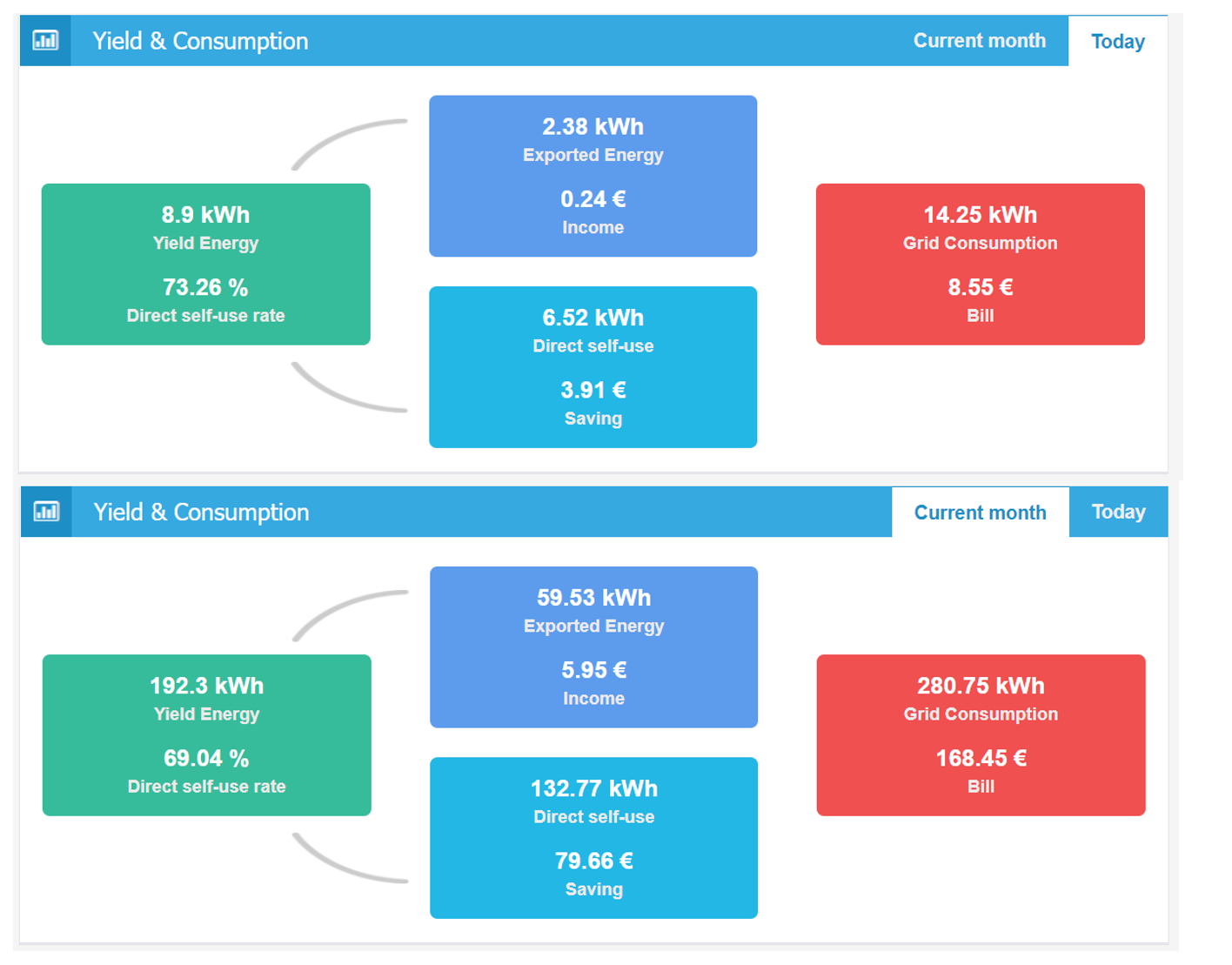# 2 Power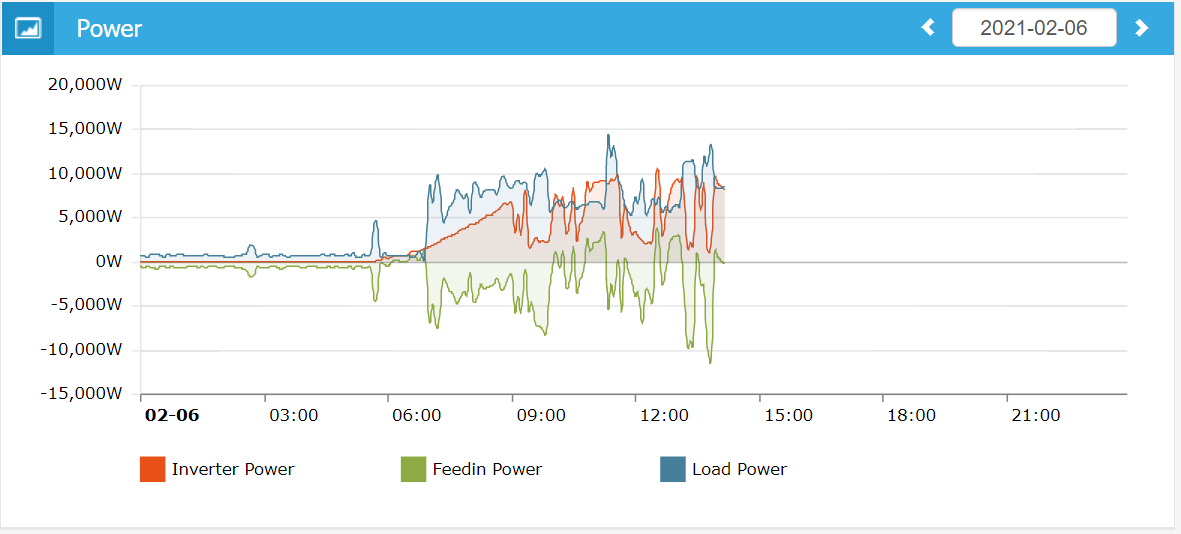**Inverter Power 😗* Output power of solar inverter,measured by the meter/CT set as "Inverter" type.

Feedin Power: It is power exporting to grid when it is positive, it is power importing from grid when it is negative, measured by the meter/CT set as "Grid" type

Load Power: Load Power = Inverter Power- Feedin Power, calculated by IAMMETER automatically.

# 3 Current state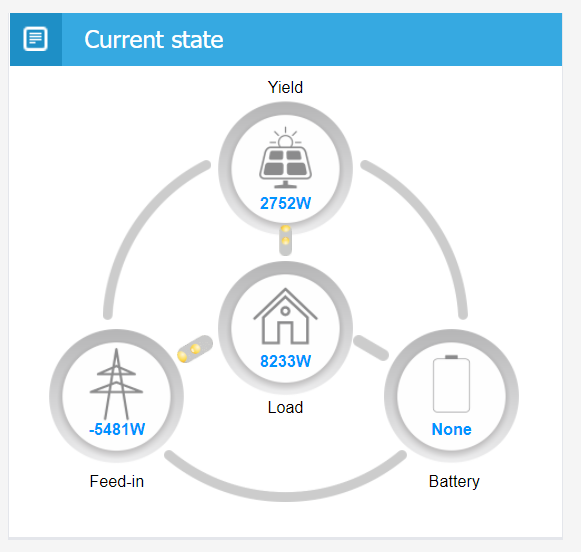This graph shows the energy flow of your solar PV system. See below coding for your better understanding.

``````var r = 0; // 0: no flow, 1:A->B, 2:B->A
switch (i)
{
case 1:// inverter-feedin
if (inverter > 0 && feedin > 0) r = 1;
break;
if (inverter > 0 && load > 0) r = 1;
break;
case 3:// inverter-battery
// the battery is charging and the inverter power is higher than load power.
if (battery > 0 && (inverter - load) > 0) r = 1;
break;
{
// if Yield is enouogh, there is no need of import energy from grid
r = 0;
break;
}
else
{
if (feedin < 0 && load > 0) r = 2;
}
break;
if (battery < 0 && load > 0) r = 2;
break;
case 6:// feedin-battery
// the battery is charging and the inverter power is lower than load power
if (feedin < 0 && (inverter - load) < battery && battery > 0) r = 1;
break;
default:
break;
}
``````

# 4 Yield

• Yield Energy :If you set any meter/CT as"Inverter" type, the energy measured by this meter/CT is "yield energy" (kWh). IAMMETER system calculates the Yield Energy Calculation in preset billing cycle. For calculation method, please refer to [Yield Energy Calculation](#Ref 2).
• Exported Energy: If you set any meter/CT as "Grid" type, the energy exported to grid measured by this meter/CT is "Exported Energy" (kWh). IAMMETER system calculates the Export Energy in preset billing cycle. For calculation method, please refer to Exported Energy Calculation
• Direct self-use: Direct self-use (kWh) = Yield Energy - Exported Energy

IAMMETER calculates the Yield Energy, Exported Energy and Direct self-use energy in the selected time period (Day, Month, Year or All Year).# 5 Consumption

• Grid Consumption: If you set any meter/CT as "Grid" type, the energy imported from grid measured by this meter/CT is "Grid Consumption" (kWh). IAMMETER system calculates the Grid Consumptionin preset billing cycle. For calculation method, please refer to Grid Consumption Calculation
• Direct self-use: Direct self-use (kWh) = Yield Energy - Exported Energy

IAMMETER calculates the Grid Consumption and Direct self-use energy in the selected time period (Day, Month, Year or All Year).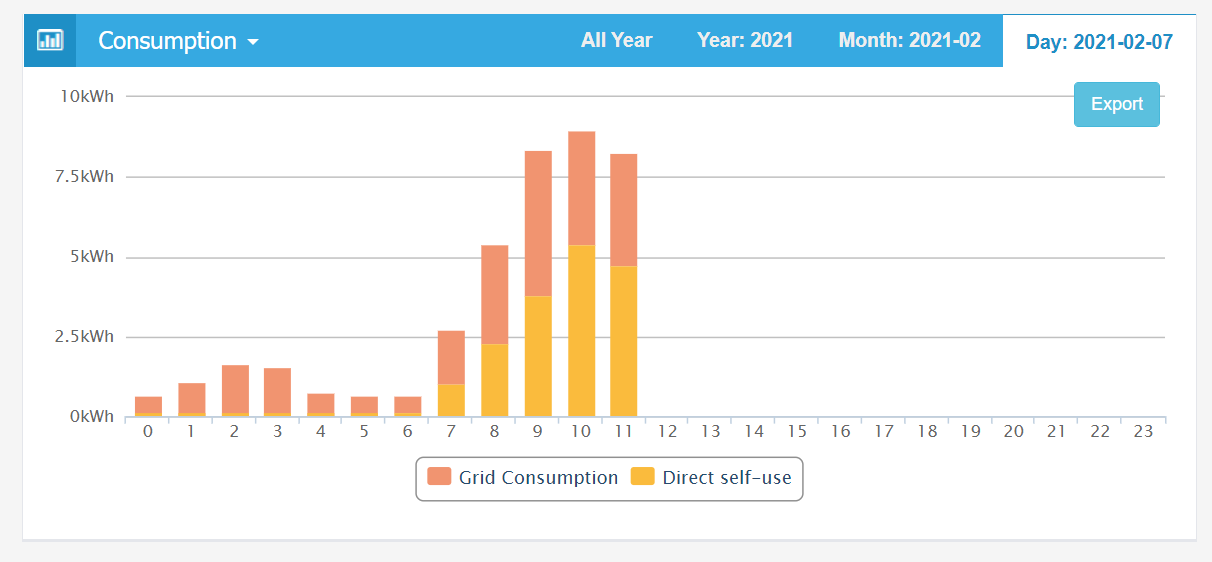# 6 Energy Balance

• Exported Energy: If you set any meter/CT as "Grid" type, the energy exported to grid measured by this meter/CT is "Exported Energy" (kWh). IAMMETER system calculates the Export Energy in preset billing cycle. For calculation method, please refer to Exported Energy Calculation
• Grid Consumption: If you set any meter/CT as "Grid" type, the energy imported from grid measured by this meter/CT is "Grid Consumption" (kWh). IAMMETER system calculates the Grid Consumption in preset billing cycle. For calculation method, please refer to Grid Consumption Calculation

IAMMETER calculates the Grid Consumption and Exported Energy in the selected time period (Day, Month, Year or All Year).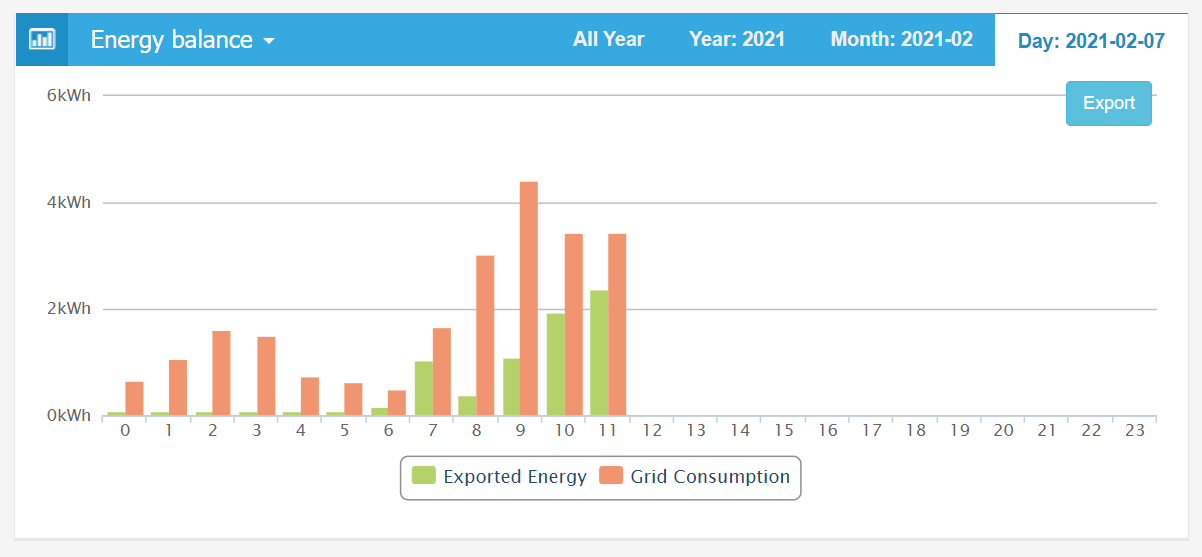# Reference

Sort and compare more than one solar PV site in the solar analysis

## Ref 1: Meter/CT type and energy, power and power tariff (import & export)

Yield Energy(kWh) & Inverter Power(W) : Meter/CT type → inverter

Export Energy(kWh) & Grid Consumption(kWh) & Feedin power(W): Meter/CT type → Grid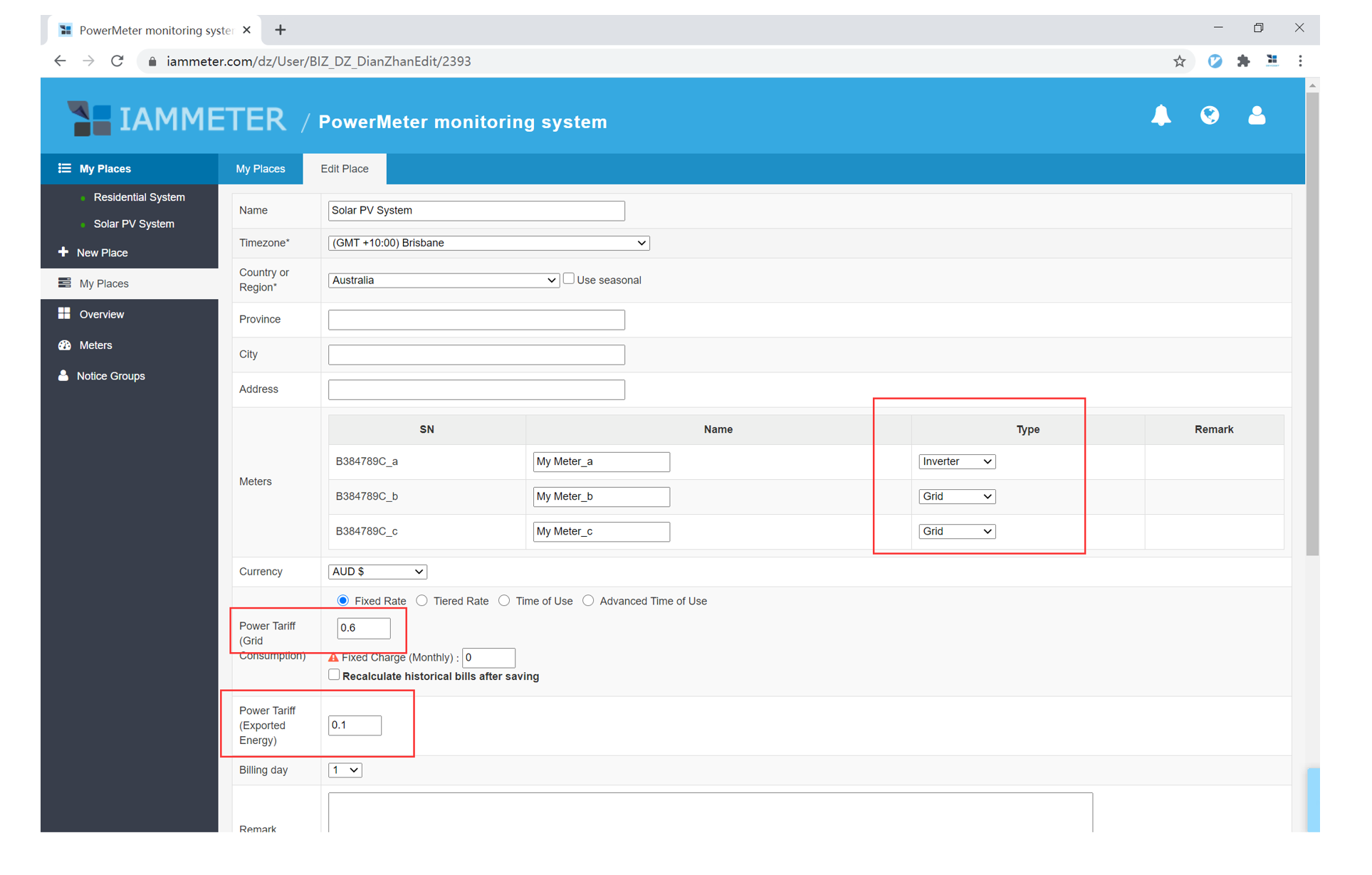## Ref 2:How IAMMETER calculates the total kWh in a selected time period (Hour, Day, Month, Year)

Total kWh = first kWh data logged in next time period - first kWh data logged in current time period

Here is a simple example. See below picture of our demo account.

On the left graph,

it shows the Total Yield Energy is 4.9kWh from 9am to 10am.

On the right table,

it shows the first kWh data logged @ 9:02:05 in current time period (9am to 10am) is 39262.5kWh,

it shows the first kWh data logged @ 10:02:06 in next time period (10am to 11am) is 39267.4kWh

39267.4 - 39262.5=4.9

So the Total Yield Energy is 4.9kWh from 9am to 10am.

The similar calculation applies to the total energy in a day, month or year.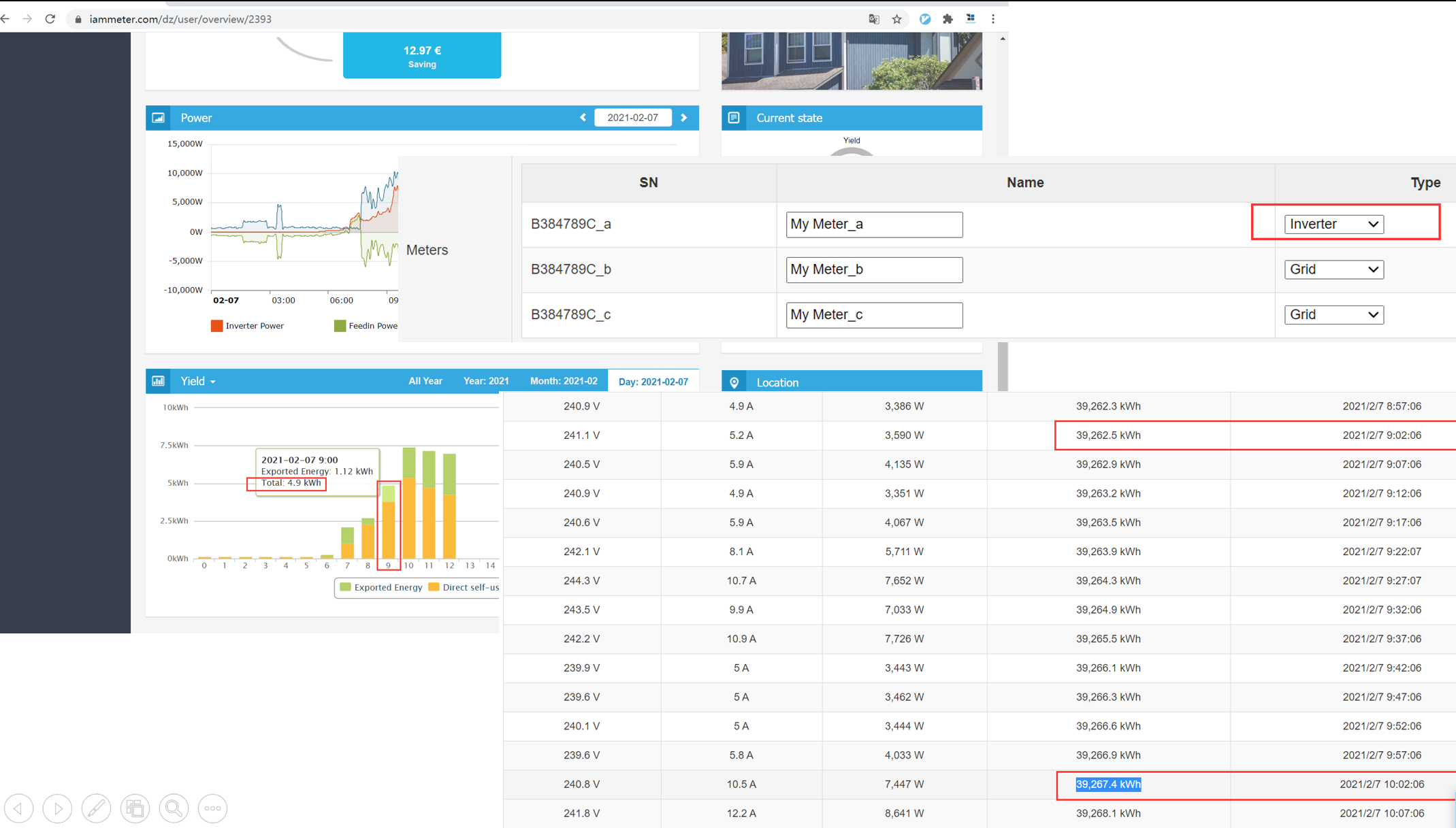## Ref 3: How IAMMETER knows monitoring a solar PV system or non-solar PV system

If you set any Meter/CT as "Inverter" type, IAMMETER identifies your system as a solar PV system.

Top